# Constructing a 45 Degree Angle

Compass and straight edge constructions are of interest to mathematicians, not only in the field of geometry, but also in algebra. For thousands of years, beginning with the Ancient Babylonians, mathematicians were interested in the problem of "squaring the circle" (drawing a square with the same area as a circle) using a straight edge and compass. This problem is equivalent to finding the area of a circle. It turns out that this is impossible, but no-one managed to prove this until 1882!

However, it is possible to construct a 45 degree angle. No cheating and getting out the protractor or set square! For this construction, you will need a straight edge (ruler - but you won't be measuring anything), pair of compasses, a pencil and paper. No cheating and getting out the protractor or set square! I have drawn the pictures using the robocompass app. It's fun to play with, and you can use it to do all sorts of geometric constructions. There's a little bit of coding to learn, but a list of instructions is provided. This construction is actually an extension of constructing the perpendicular bisector of a straight line, so the program is a bit longer than some of the other ones we've used. It still probably isn't worth getting out the popcorn, but you can sit back for a minute or so to watch the construction. Be warned that, when you are using it to draw arcs, the robocompass compass point may appear to be a little away from the centre, but the drawing is actually accurate.

Let's start by reviewing the construction of the perpendicular bisector. Feel free to skip this bit if you feel you are an expert on this construction.

## Constructing the Perpendicular Bisector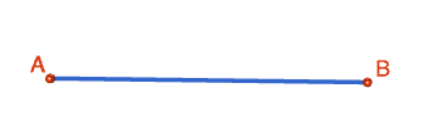Step 1: Start out by drawing the line segment $AB$ that you want to bisect. Please don't be like Sam! Use a ruler to draw this.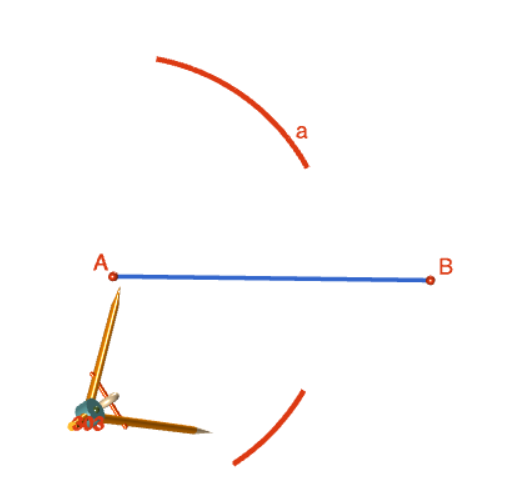Step 2: Place the tip of your pair of compasses on point $A$, and open them out to about $\dfrac{2}{3}$ of the way along your line segment. You are going to draw four arcs of this radius, so make sure you keep it fixed. Draw two arcs (one above and one below the line segment). The picture shows the tip of the compass a little away from point $A$. This is just a problem with the animation. The arcs are actually centred at point $A$, so make sure your compass tip is exactly on point $A$.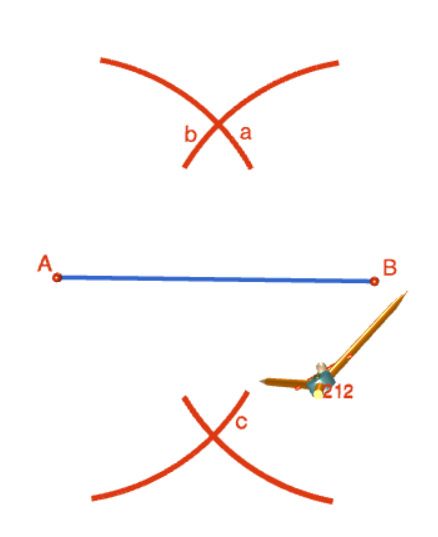Step 3: Keeping your compasses at the same radius as in the preceding step, place the tip of your pair of compasses on point $B$. Draw two arcs (one above and one below the line segment). The picture shows the tip of the compass a little away from point $B$. This is just a problem with the animation. The arcs are actually centred at point $B$, so make sure your compass tip is exactly on point $B$. As shown in the picture, your arcs need to intersect the arcs drawn in step 2.Step 4: Use your ruler to join the points of intersection of both pairs of arcs (above and below the line).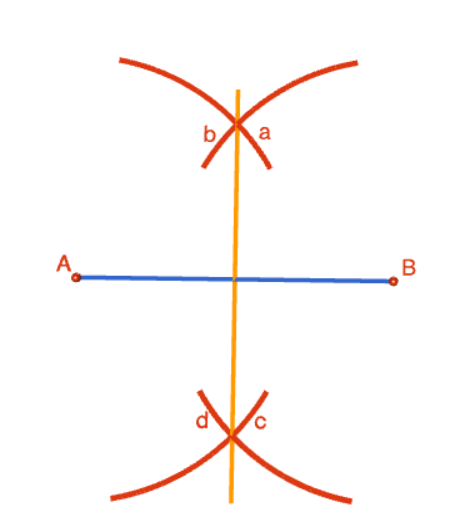This is the finished product: a bisected straight line. You can rub the arcs out, if you like. The vertical line (shown in orange) is at right angles to line segment $AB$, and cuts it in half. Now you can do some measuring and use your ruler to check!

## Building that 45 Degree Angle

Now we have just a couple more steps to complete so that we can build our 45 degree angle. I've actually used a different bisected line here as I needed a bit more room to add in another arcs. I made the radius of the arcs a little smaller than in the original construction.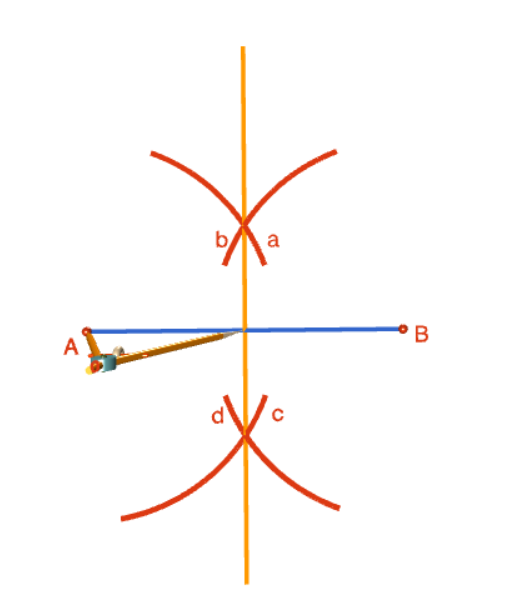Step 5: Put the tip of your compass at point A and open the compass out so that the pencil is on the point of intersection between the straight line and its bisector. Don't draw anything. We're just getting the right radius.Step 6: Now it's time to draw an arc. Keeping the radius found in the preceding step, move the tip of your compass to the point of intersection between the straight line and its bisector. Draw an arc that passes through the top half of the bisector. Label the point of intersection of your arc with the bisector $C$.Step 7: Now we'll draw the second arm of our angle. Get out your straight edge (please!) and draw a line segment joining point $A$ to point $C$. We're finished! Now you can dig out your protractor and measure the angle to make sure everything went according to plan. Of course, you can erase all those messy arcs and perpendicular bisectors if you like.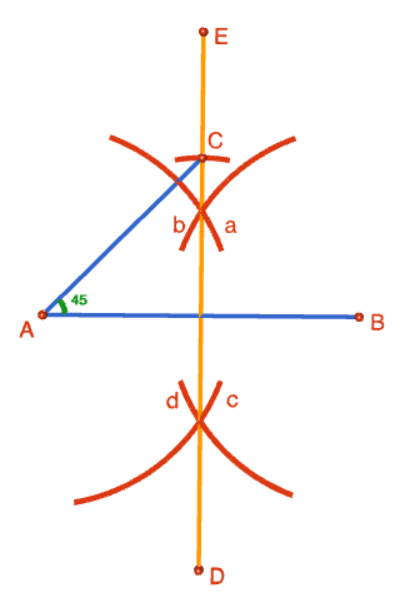### Description

This tutorial will expose you to Rulers and how to use them. We will also have a close look as set square and compass constructions. Your feedback is important to us, if you like any other topic covered under this tutorial, please do let us know.

### Audience

Year 10 or higher

### Learning Objectives

Ruler and compass constructions and more

Author: Subject Coach
You must be logged in as Student to ask a Question.